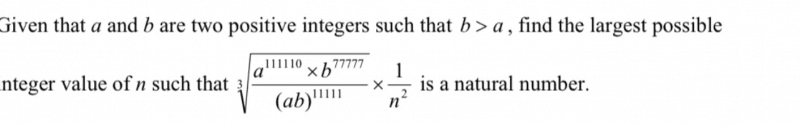# Question∛[(a111110 × b77777) / (ab)11111] × (1 / n2)
= ∛(a99999 × b66666) × (1 / n2)
= a33333 × b22222 × (1 / n2)
= a11111 × a22222 x b22222 × (1 / n2)
= a11111 × (a11111)2 × (b111111)2 × (1 / n2)
= a × (a5555)2 × (a11111)2 × (b111111)2 × (1 / n2)
= a × (a5555 × a11111 × b111111)2 × (1 / n2)
= a × (a16666 × b11111)2 × (1 / n2)
= [a × (a16666 × b11111)2] / n2

Since “n” must be as large as possible, this means that n2 = (a16666 × b11111)2, so that they cancel each other out and still leave “a” as a positive integer. The largest possible value of “n” is therefore: a16666× b1111.

0 Replies 0 Likes